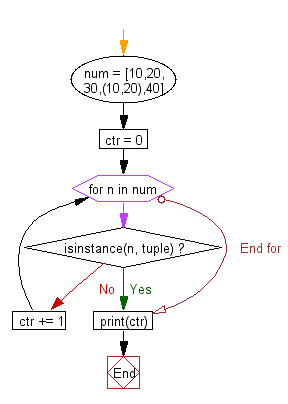﻿ Python: Count the elements in a list until an element is a tuple - w3resource# Python Exercise: Count the elements in a list until an element is a tuple

## Python tuple: Exercise-24 with Solution

Write a Python program to count the elements in a list until an element is a tuple.

Sample Solution:-

Python Code:

``````num = [10,20,30,(10,20),40]
ctr = 0
for n in num:
if isinstance(n, tuple):
break
ctr += 1
print(ctr)
```
```

Sample Output:

```3
```

Flowchart:## Visualize Python code execution:

The following tool visualize what the computer is doing step-by-step as it executes the said program:

Python Code Editor:

Have another way to solve this solution? Contribute your code (and comments) through Disqus.

What is the difficulty level of this exercise?

Test your Python skills with w3resource's quiz

﻿

## Python: Tips of the Day

Returns the maximum value of a list, after mapping each element to a value using the provided function

Example:

```def tips_max(lst, fn):
return max(map(fn, lst))

print(tips_max([{ 'n': 4 }, { 'n': 2 }, { 'n': 8 }, { 'n': 6 }], lambda v : v['n']))
```

Output:

```8
```